## Equilibrium in Physical Processes

Physical process involves such changes, which only affects the physical properties of the substance undergoing changes but have no effect on the chemical composition and properties.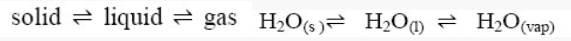### Solid-Liquid Equilibrium:

The equilibrium is represented as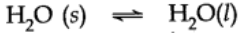Rate of melting of ice = Rate of freezing of water.
The system here is in dynamic equilibriums and following can be inferred.

• Both the opposing processes occur simultaneously.
• Both the processes occur at the same rate so that the amount of ice and water – remains constant.

### Liquid-Vapour Equilibrium

The equilibrium can be represented as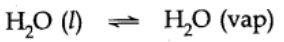Rate of evaporation = Rate of condensation
When there is an equilibrium between liquid and vapours, it is called liquid-vapour equilibrium.

### Solid-Vapour Equilibrium

This type of equilibrium is attained where solids sublime to vapour phase. For example, when solid iodine is placed in a closed vessel, violet vapours start appearing in the vessel whose intensity increases with time and ultimately, it becomes constant.

The equilibrium may be represented as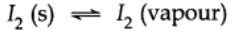Rate of sublimation of solid I2 to form vapour = Rate of condensation of I2 vapour to give solid I2

## Equilibrium in Chemical Processes

Chemical processes involve changes in chemical composition and properties. Whenever a chemical change occurs, we can say that a chemical reaction has taken place.

For example,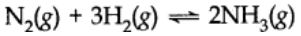The dynamic nature of chemical equilibrium can be demonstrated in the synthesis of ammonia by Haber’s process. Haber started his experiment with the known amounts of N2 and H2 at high temperature and pressure. At regular intervals of time he determined the amount of ammonia present. He also found out concentration of unreacted N2 and H2.
After a certain time he found that the composition of mixture remains the same even though some of the reactants are still present. This constancy indicates the attainment of equilibrium. In general, for a reversible reaction the chemical equilibria can be shown by

A + B ⇌ C + B

After a certain time, the two reactions occur at the same rate and the system reaches a state of equilibrium. This can be shown by the given figure.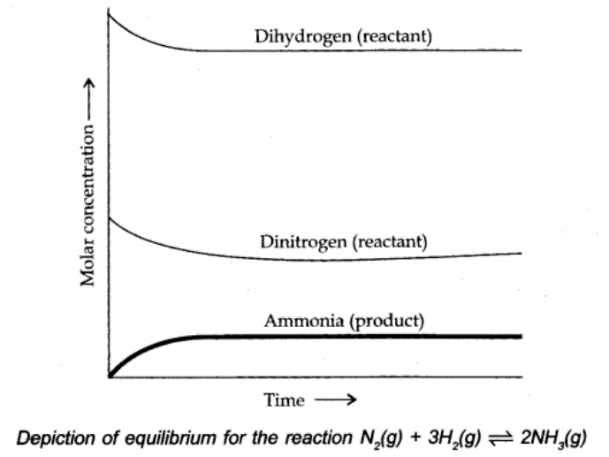## Reversible Reaction

A reaction in which not only the reactants react to form the products under certain conditions but also the products react to form reactants under the same conditions is called a reversible reaction.

In other word a reaction which takes place not only in the forward direction but also in the backward direction under the same conditions is called a reversible reaction.

A reversible reaction between A and B to form C and D is represented as:

A + B ⇌ C + B

## Irreversible Reactions

If a reaction cannot take place in the reverse direction, i.e., the products formed do not react to give back the reactants under the same conditions, it is called an irreversible reaction.

A + B ⟶ C + D